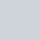# Most Tricky GATE Questions on Recursion

Last updated on

Are you a GATE aspirant?

And, searching for the step by step solution✍ on recursion?

Here, I came up with the most tricky GATE questions on recursion.

From the previous years’ questions, I picked up the most tricky ones with step-by-step clarification to get a solid grip on recursion.

Let’s get started.

### Question 1: (GATE 2005: 2 Marks)

What is the output printed by the following program?

``````#include<stdio.h>
int f(int n, int k)
{
if(n==0) return 0;
else if(n%2) return f(n/2, 2*k)+k;
else return f(n/2, 2*k)-k;
}
int main()
{
printf("%d",f(20,1));
return 0;
}``````
1. 5
2. 8
3. 20

### Question 2: (GATE 2007: 2 Marks)

Consider the following C function:

``````int f(int n)
{
static int r=0;
if(n<=0) return 1;
if(n>3)
{
r=n;
return f(n-2)+2;
}
return f(n-1)+r;
}``````

What is the value of f(5)?

1. 5
2. 7
3. 9

### Question 3: (GATE 2008: 2 Marks)

Consider the code fragment written in C below:

``````void f(int n)
{
if(n<=1)
{
printf("%d",n);
}
else
{
f(n/2);
printf("%d",n%2);
}}``````

What does f(173) print?

• 010110101
• 010101101
• 10110101

### Question 4: (GATE 2011: 2 Marks)

Consider the following recursive C function that takes two arguments.

``````unsigned int foo(unsigned int n, unsigned int r)
{
if(n>0) return((n%r)+foo(n/r,r));
else return 0;
}``````

What is the return value of the function foo when it is called as foo(345,10)?

1. 345
2. 5
3. 3

### Question 5: (GATE 2011: 2 Marks)

Consider the following recursive C function that takes two arguments.

``````unsigned int foo(unsigned int n, unsigned int r)
{
if(n>0) return((n%r)+foo(n/r,r));
else return 0;
}``````

What is the return value of the function foo when it is called as foo(513,2)?

• 9
• 8
• 5

## Final Words

If you’re a CS GATE aspirant, it’s very important to have a clear concept on various topics like recursion.

Sometimes, it becomes very difficult to find a step-by-step solution to a program. So, I tried to help you to solve the GATE questions on recursion.

Hope, you liked this post, and if you’ve any related suggestions or query, feel free to let me know.👇

### 3 responses to “Most Tricky GATE Questions on Recursion”

1.AVISHEK CHATTERJEE

Great work , thanks for such simple solutions . Love it ..

2.Prashant

What a great content, really now I am able to understand how to start solving recursion questions.
Thanks brothers

3.Umamaheswari

Thanks a lot, very useful 👍